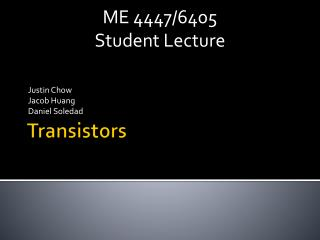DownloadDownload PresentationTransistors

# Transistors

Download Presentation## Transistors

- - - - - - - - - - - - - - - - - - - - - - - - - - - E N D - - - - - - - - - - - - - - - - - - - - - - - - - - -
##### Presentation Transcript

1. ME 4447/6405 Student Lecture Justin Chow Jacob Huang Daniel Soledad Transistors

2. Overview • History • Properties • Types • BJT • JFET • MOSFET • Applications Daniel Soledad

3. Introduction • Transistor History • “Transistor” is combination of “transconductance” and “variable resistor” • How Transistors Are Made • Vacuum tubes • Inefficient, fragile, bulky, generated a lot of heat • First Transistors • Semiconductors – Bell Labs 1947

4. Introduction • Packaging • Surface Mount or Through Hole • Usually 3 or 4 terminal device • Can be packaged into ICs • General Applications • Amplification /Regulation • Switches

5. General Operation • Current Controlled • i.e: BJT • The output current is proportional to input current • Voltage Controlled • i.e.: JFET, MOSFET • The output current is proportional to input voltage

6. BJT Transistor Justin Chow • Bipolar Junction Transistor • 3 semiconductor layers sandwiched together • Comes in two flavors NPN BJT PNP BJT

7. BJT Transistor • Diodes Forward Biased Reverse Biased current flows no current flows when VPN > .6-.7V

8. emitter base collector Electron Flow BJT Transistor • BJT Basics (NPN) • BE Forward Biased • BC Reversed Biased • β=IB / Ic ≈ 100 • IE = IB + IC

9. BJT Transistor • Things to remember • PNP, biasing opposite • Conventional current vs electron flow • A small input current controls a much larger output current.

10. BJT Transistor Operating Regions

11. BJT Transistor Operating Regions

12. Application: Switching • From 3rd Exercise • Turns on/off coils digitally

13. BJT Transistor β=100 Common Emitter Amplifier

14. BJT Transistor • IB = (Vin − VB) / 10000Ω = (Vin − 0.7) / 10000Ω • IC = β(Vin − 0.7) / 10000Ω • Vout=10000*(Vin-0.7)/1000 • When VCE = 0.2V • IC = 9.8 / 1000Ω = 9.8mA • IB = IC / β = 0.098mA • Vin − 0.7 = (0.098mA)(10000Ω) • Vin = 1.68V or greater. Common Emitter Amplifier

15. BJT Transistor • Power Dissipation • PBJT = VCE * iCE • Should be below the rated transistor power • Important for heat dissipation as well

16. Darlington Transistors Increased Gain β = β1 * β2 VBE = VBE1 + VBE2 Slower Switching 2N6282

17. FET Transistors Analogous to BJT Transistors Output is controlled by input voltage rather than by current 4 Pins vs. 3

18. FET Transistors FET (Field Effect Transistors) MOSFET (Metal-Oxide-Semiconductor Field-Effect Transistor) JFET (Junction Field-Effect Transistor) MESFET HEMT MODFET Most common are the n-channel MOSFET or JFET Jacob Huang

19. FET Transistors – Circuit Symbols In practice the body and source leads are almost always connected Most packages have these leads already connected D D D B G G B G S S S MOSFET JFET

20. MOSFET Metal-Oxide Semiconductor F.E.T. A.K.A. Insulated-Gate FET (IGFET) 2 Modes: Enhancement/Depletion

21. Depletion Mode • N-Channel • + Vgs -> More electrons -> More Current • - Vgs -> Less electrons -> Less current • P-Channel – Reversed • Different from BJT

22. Characteristic Curves for D-type D G B S Current flow

23. Enhancement Mode • N-Channel • VGS > Vth -> Turns on device • VGS < VTH -> No Current • P-Channel • Reversed • Only E-type used now

24. Modes of Operation D G B S Current flow

25. Characteristic Curve for E-type D G B S Current flow

26. Power MOSFET • Used in high-power applications • Heat Sink • Vertical layout • Not Planar like other transistors

27. Junction gate FET Reverse Bias VGS => Reduces channel size => Reduced Current Defaults “on”

28. JFET as Switch Vgs = 0 “on” |Vgs|> |Vp| “off” Vp = Pinch-off or Cut-off Voltage

29. JFET Properties • Internal Capacitance • Bi-directional • Cut-off voltage is varying for each JFET • 0.3V – 10V • N-Channel – Negative VGS • P-Channel – Positive VGS • Do not Forward Bias JFET – burn out

30. JFET Characteristic Curve

31. Comparison

32. CMOS Complementary MOS Used in Logic Gates P-channel (PMOS) to high N-channel (NMOS) to low HIGH usually +5 V LOW usually ground Q is high when A = 0, Q is low when A = 1